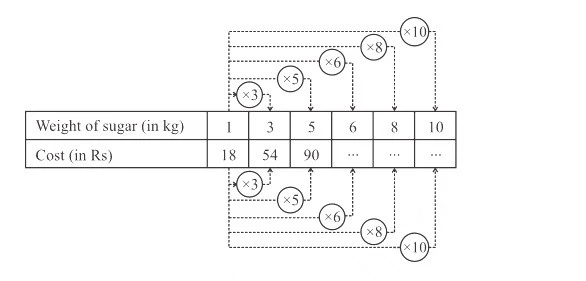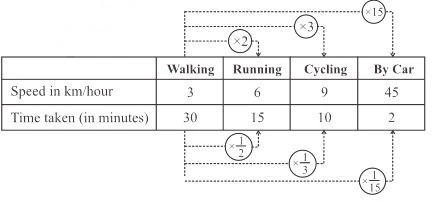or# Direct And Inverse Proportions NCERT Solutions : Class 8 Maths

## NCERT Solutions for Class 8 Maths : Direct And Inverse ProportionsFilter
Filters :
Classes
•
•
•
•
•
•
•
Chapters
•
•
•
•
•
•
•
•
•
•
• 5 More
Exercises
•
•
•

### NCERT Class 8 | DIRECT AND INVERSE PROPORTIONS | Exercise 02 | Question No. 10

Two persons could fit new windows in a house in 3 days. (i)            One of the persons fell ill before the work started. How long would the job take now? (ii)           How many persons would be needed to fit the windows in one day?Doubtnut one of the best online education platform provides free NCERT Solutions of Maths for Class 8 Direct And Inverse Proportions which are solved by our maths experts as per the NCERT (CBSE) guidelines. We provide the solutions as video solutions in which concepts are also explained along with answer which will help students while learning , preparing for exams and in doing homework. These Solutions will help to revise complete syllabus and score more marks in examinations.

Get here free, accurate and comprehensive NCERT Solutions for Class 8 Maths Direct And Inverse Proportions which have been reviewed by our maths counsellors as per the latest edition following up the CBSE guidelines. We provide video solutions in which solutions to all the questions of NCERT Class 8 Maths textbook are explained in step by step and detailed way.

## The Topics of the Chapter Direct And Inverse Proportions are :

APPLICATION OF LINEAR EQUATIONS TO PRACTICAL PROBLEMS

TYPES OF VARIATIONS

INTRODUCTION

VARIATIONS

INVERSE VARIATION

INTRODUCTION TO TIME AND WORK

DIRECT VARIATION

It contains these exercises along with solved examples. All the exercises are solved in the video. Select the exercise to view the solutions exercisewise of the Chapter Direct And Inverse Proportions:

## NCERT Solutions Class 8 Maths Chapter Direct And Inverse Proportions Exercises:

We have covered all the exercises and also Solved examples in the videos. Along with the practise exercise Students should also practise solved examples to clear the concepts of the Direct And Inverse Proportions. If incase you have any doubt you can watch the solutions for the given questions.Watch the solutions for the given questions in which it is also explained in the video steps to solve the questions along with answers.

# NCERT Class 8 Maths Solutions Chapter 13 Direct and Inverse Proportions

This chapter focuses on concepts of variation between two variables. In this chapter you will be made familiar with the relation between two variables that determines the change in the value of one variable when the value of the other variable is changed. Here, students are able to get the best idea on its applications and properties. It also includes the concept right from the alpha to omega to deal with direct as well as inverse proportions. With daily life examples, it becomes possible for the students to get in depth understanding of the topic. The expert team of Doubtnut has been able to cover all the topics in the best and simple manner. So, students do not find it any sort of difficulty in understanding the subject matter.

The NCERT books help in understanding the basic concepts of the subject with adequate examples and theoretical explanations. It is observed that most of the questions asked in CBSE examinations are from the NCERT book. The NCERT book provides precise theory and also quality questions for effective practice. But in order to get comprehensive knowledge of the chapter, you must practice the NCERT book rigorously. NCERT maths book contains solved examples and unsolved exercise questions. For self-study, you need the solutions of unsolved exercises to confirm your approach and result.  For that purpose, you either seek for a solution guide or look for a tutor to help you out with solutions. Both the options require much time and money.  Here, Doubtnut provides free NCERT maths class 8 solutions, so that the students need not to wander here and there for different reference books for NCERT solutions or paying hefty amount to the tutors.

At Doubtnut, we make sure that the solution is presented in easy to understand and detailed manner.  The solutions are made in the most preferred manner so that any students either novice or someone who is familiar both can be benefited. The subject matter experts at Doubtnut have made the best effort to provide online study materials based on different pace of learning. These video tutorials for NCERT Class 8 Maths Solutions Chapter 13 provided the students with immersive and effective learning experience. This is because of the step-by-step procedure followed in the solutions. It is also possible to download the NCERT solutions in pdf form. So, this helps the students to prepare for their exams offline. So, it truly helps them to clear all their doubts for which they even solve the most complicated questions with ease. Also, the most helpful Class 8 Maths NCERT Solutions provided by them adds to the advantages of the students. It is also possible for them to have the complete control of the video tutorials where they can rewind, fast-forward, etc.

## Topics and Subtopics included in Class 8 Maths Chapter 13 Direct and Inverse Proportions

### Introduction

There are many situations in our day-to-day life where we can find variation in one quantity that brings variation in the other quantity.  Some of the examples that can be seen are:

• If you deposit more money, more interest can be earned.
• If you drive your car in a good speed, it takes less time in covering the distance.
• More number of workers helps to complete a huge task in a much lesser time.

Thus, it can be seen that if there is change in the value of one entity, it also leads to change in the value of the other entity.

### Direct Proportion

Suppose the price of 1 kg of sugar is Rs 18, then what would be the price of 3kg sugar? The answer is simple which is Rs 54.It can be observed that if there is an increase in the weight of sugar, then cost also increases, whereas the ratio of weight and cost remains constant.

Let’s take one more example. Suppose a car need 4 litres of petrol to reach a particular destination which is 60 km. Now can you tell how far can the car travel with 12 litres? It is 180 km. No how did we find out the answer? In the second instance, since petrol consumed is 12 litres which is three times of 4 litres, it becomes three times of 60 km for the distance travelled. Thus it can be said that both the petrol and the distance traveled would be three-fold.  If we assume that the consumption of petrol is x litres and y is the distance traveled then x and y are in direct proportion. So, x/y =k or x=ky. The distance travelled by a car and the petrol consumption is a case of direct proportion.

### Inverse Proportion

It is to be noted that there are instances where changes might occur in two quantities in such a manner that if there is an increase in one quantity there is a decrease in the other quantity and vice versa.  For example time taken to finish a job decreases when there is an increase in the number of workers.

For example: Rohan can go to school in four different ways – walking, running, cycling or by car. Check the table:It can be clearly seen that there is a decrease in the duration of the time with the increase of speed. Time decreases to one third with the increase of her speed to three times by cycling.  Hence, the ratio of decrease in time is inverse of the ratio with increase of the corresponding speed.

## Role of Doubtnut for Class 8 Maths students

Doubtnut has made a significant place for itself in e-learning domain.  With the introduction of the free video tutorials and swift doubt clearance, Doubtnut is helping the students in building concrete academic foundation leading to a bright future. There are other advantages of learning with doubtnut:

• Distraction-free learning environment:  Doubtnut provides solutions in video format and pdf format. You can download the solutions to study and learn from them at the convenient time and place. In this way, you can fully concentrate on a subject like Maths that truly requires lots of concentration. By studying in a noise-free room, it helps a lot to get all the queries cleared in the right manner.
• Get 24x7 access to the study materials:  It also does not matter whether you are at home or even travelling, you can always get access to the online study material. This can help a lot to enjoy flexibility in your studies. Therefore, students can really get hold of all the right details on how to clear their queries in the comfort of their home.
• Free of cost: Another added advantage that the students can enjoy by opting for the online study materials is that they do not have to pay any money to access it. This proves to be quite useful and profitable for them where they do not have to look elsewhere to get their queries cleared. Therefore, making the most important and best decision to opt for Doubtnut’s online video tutorials can really help them in achieving success in the examinations.